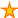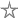# Rounding in Access

6 votes:6,633 views      No comments
by Allen Browne, 18 January 2008    (for Access 95+)

### Microsoft Access Tips for Casual Users

Provided by Allen Browne.  Created: December 2007.  Updated August 2008.

# Rounding in Access

To round numbers, Access 2000 and later has a Round() function built in.
For earlier versions, get this custom rounding function by Ken Getz.

## The built-in function

Use the Round() function in the Control Source of a text box, or in a calculated query field.

Say you have this expression in the Field row in query design:
Tax: [Amount] * [TaxRate]
To round to the nearest cent, use:
Tax: Round([Amount] * [TaxRate], 2)

## Rounding down

To round all fractional values down to the lower number, use Int():
Int([MyField])

All these numbers would then be rounded down to 2: 2.1, 2.5, 2.8, and 2.99.

To round down to the lower cent (e.g. \$10.2199 becomes \$10.21), multiply by 100, round, and then divide by 100:
Int(100 * [MyField]) / 100

Be aware of what happens when negative values are rounded down: Int(-2.1) yields -3, since that is the integer below. To round towards zero, use Fix() instead of Int():
Fix(100 * [MyField]) / 100

## Rounding up

To round upwards towards the next highest number, take advantage of the way Int() rounds negative numbers downwards, like this:
- Int( - [MyField])

As shown above, Int(-2.1) rounds down to -3. Therefore this expression rounds 2.1 up to 3.

To round up to the higher cent, multiply by -100, round, and divide by -100:
Int(-100 * [MyField]) / -100

## Round to nearest 5 cents

To round to the nearest 5 cents, multiply the number by 20, round it, and divide by 20:
Round(20 * [MyField], 0) / 20

Similarly, to round to the nearest quarter, multiply by 4, round, and divide by 4:
Round(4 * [MyField], 0) / 4

## Round to \$1000

The Round() function in Excel accepts negative numbers for the number of decimal places, e.g. Round(123456, -3) rounds to the nearest 1000. Unfortunately, the Access function does not support this.

To round to the nearest \$1000, divide by 1000, round, and multiply by 1000. Example:
1000 * Round([Amount] / 1000, 0)

To round down to the lower \$1000, divide by 1000, get the integer value, and multiply by 1000. Example:
1000 * Int([Amount] / 1000)

To round up to the higher \$1000, divide by 1000, negate before you get the integer value. Example:
-1000 * Int( [Amount] / -1000)

To round towards zero, use Fix() instead of Int().

Alternatively, Ken Getz' custom rounding function behaves like the Excel function.

## Why round?

There is a Decimal Places property for fields in a table/query and for text boxes on a form/report. This property only affects the way the field is displayed, not the way it is stored. The number will appear to be rounded, but when you sum these numbers (e.g. at the foot of a report), the total may not add up correctly.

Round the field when you do the calculation, and the field will sum correctly.

This applies to currency fields as well. Access displays currency fields rounded to the nearest cent, but it stores the value to the hundredth of a cent (4 decimal places.)

## Bankers rounding

The Round() function in Access uses a bankers rounding. When the last significant digit is a 5, it rounds to the nearest even number. So, 0.125 rounds to 0.12 (2 is even), whereas 0.135 rounds to 0.14 (4 is even.)

The core idea here is fairness: 1,2,3, and 4 get rounded down. 6,7,8, and 9 get rounded up. 0 does not need rounding. So if the 5 were always rounded up, you would get biased results - 4 digits being rounded down, and 5 being rounded up. To avoid this, the odd one out (the 5) is rounded according to the previous digit, which evens things up.

If you do not wish to use bankers rounding, get Ken Getz' custom function (linked above.)

## Floating point errors

Fractional values in a computer are typically handled as floating point numbers. Access fields of type Double or Single are this type. The Double gives about 15 digits of precision, and the Single gives around 8 digits (similar to a hand-held calculator.)

But these numbers are approximations. Just as 1/3 requires an infinite number of places in the decimal system, most floating point numbers cannot be represented precisely in the binary system. Wikipedia explains the accuracy problems you face when computing floating point numbers.

The upshot is that marginal numbers may not round the way you expect, due to the fact that the actual values and the display values are not the same. This is especially noticeable when testing bankers rounding.

One way to avoid these issues is to use a fixed point or scalar number instead. The Currency data type in Access is fixed point: it always stores 4 decimal places.

For example, open the Immediate Window (Ctrl+G), and enter:
? Round(CCur(.545),2), Round(CDbl(.545),2)
The Currency type (first one) yields 0.54, whereas the Double yields 0.55. The Currency rounds correctly (towards the even 4); the floating point type (Double) is inaccurate. Similarly, if you try 8.995, the Currency correctly rounds up (towards the even 0), while the Double rounds it down (wrong.)

Currency copes with only 4 decimal places. Use the scalar type Decimal if you need more places after the decimal point.

## Rounding dates and times

Note that the Date/Time data type in Access is a special kind of floating point type, where the fractional part represents the time of day. Consequently, Date/Time fields that have a time component are subject to floating point errors as well.

The function below rounds a date/time value to the specified number of seconds. For example, to round to the nearest half hour (30 * 60 seconds), use:
=RoundTime([MyDateTimeField], 1800)

```Public Function RoundTime(varTime As Variant, Optional ByVal lngSeconds As Long = 900&) As Variant
'Purpose:   Round a date/time value to the nearest number of seconds
'Arguments: varTime = the date/time value
'           lngSeconds = number of seconds to round to.
'               e.g.  60 for nearest minute,
'                    600 for nearest 10 minutes,
'                   3600 for nearest hour,
'                  86400 for nearest day.
'Return:    Rounded date/time value, or Null if no date/time passed in.
'Note:      lngSeconds must be between 1 and 86400.
'           Default rounds is nearest 15 minutes.
Dim lngSecondsOffset As Long

RoundTime = Null        'Initialize to return Null.
If Not IsError(varTime) Then
If IsDate(varTime) Then
If (lngSeconds < 1&) Or (lngSeconds > 86400) Then
lngSeconds = 1&
End If
lngSecondsOffset = lngSeconds * CLng(DateDiff("s", #12:00:00 AM#, TimeValue(varTime)) / lngSeconds)
End If
End If
End Function```

## Conclusion

For a more technical explanation of rounding theory in general, see Clive Maxfield's article, An introduction to different rounding algorithms.

Rate this article:This is a cached tutorial, reproduced with permission.